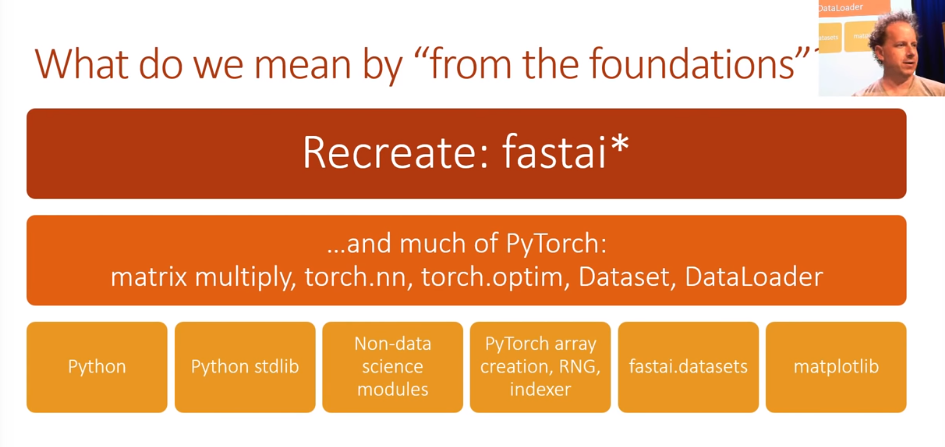# Deep Learning from Foundations

Source: Deep Learning on MediumForward and Backward pass

`def normalize(x, m, s): return (x-m)/strain_mean,train_std = x_train.mean(),x_train.std()train_mean,train_std(tensor(0.1304), tensor(0.3073))`

The mean and std are not 0 and 1, However we want them to be 0 or 1. Hence we apply the normalization function

`x_train = normalize(x_train, train_mean, train_std)# NB: Use training, not validation mean for validation setx_valid = normalize(x_valid, train_mean, train_std)train_mean,train_std = x_train.mean(),x_train.std()train_mean,train_std(tensor(3.0614e-05), tensor(1.))# mean and std now are much closer to 1n,m = x_train.shapec = y_train.max()+1 # Number of activation's or outputsn,m,c(50000, 784, tensor(10))`

Now lets Try to create model with 1 hidden layer. For simplification we will use MSE for the time being.

We create a hidden layer with number of neurons = 50 . For 2 layers we shall need 2 weight matrices and 2 bias

`# num hiddennh = 50# simplified kaiming init / he initw1 = torch.randn(m,nh)/math.sqrt(m)b1 = torch.zeros(nh)w2 = torch.randn(nh,1)/math.sqrt(nh)b2 = torch.zeros(1)test_near_zero(w1.mean())test_near_zero(w1.std()-1/math.sqrt(m))`

We have input x_valid( input to layer 1 ) with mean 0 and std 1 , we want input to second layer to also be of mean 0 and std 1.But if we divide by math.sqrt(m) we achieve the same. This is a simplified version of kaiming initialization.

`# This should be ~ (0,1) (mean,std)...x_valid.mean(),x_valid.std()(tensor(-0.0058), tensor(0.9924))def lin(x, w, b): return x@w + bt = lin(x_valid, w1, b1)#...so should this, because we used kaiming init, which is designed to do thist.mean(),t.std()(tensor(0.0004), tensor(0.9786))`

torch.randn(m,nh) gives a mean of 0 and std of 1 and torch.randn(m,nh)/math.sqrt(m) gives std of 1/sqrt(m)

Careful initialization is key for good NN performance

`t = lin(x_valid, w1, b1) # is not how first layer defined`

Initial layer is defined by relu.

`def relu(x): return x.clamp_min(0.)t = relu(lin(x_valid, w1, b1))#...actually it really should be this!t.mean(),t.std()(tensor(0.3875), tensor(0.5665))`

Post relu the out put does not have mean 0 and std 1. This was solved by kaiming initialization.